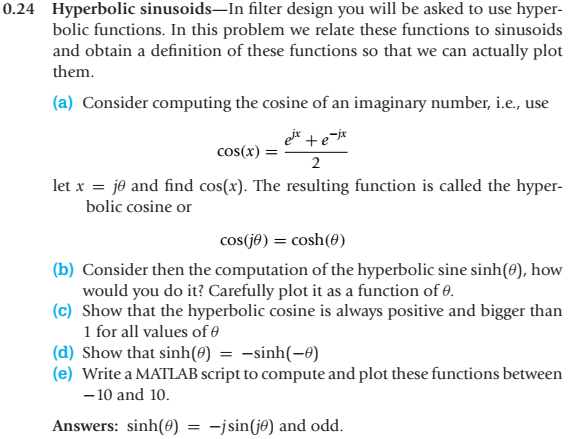1

## Question

Please add comments line for matlab and show all step in paper, ASAP. Thanks in advance.

0.24 Hyperbolic sinusoids-In filter design you will be asked to use hyper bolic functions. In this problem we relate these functions to sinusoids and obtain a definition of these functions so that we can actually plot them (a) Consider computing the cosine of an imaginary number, i.e., use cos(x) 2 let j and find cos(x). The resulting function is called the hyper- bolic cosine or cos(je) = cosh(0) b) Consider then the computation of the hyperbolic sine sinh(0), how would you do it? Carefully plot it as a function of e. (c) Show that the hyperbolic cosine is always positive and bigger than 1 for all values of 0 d) Show that sinh(e)-sinh(-0) e) Write a MATLAB script to compute and plot these functions between -10 and 10 Answers: sinh(0) -j sin(je) and odd.